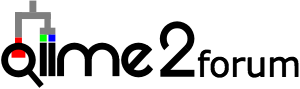# Levels in Songbird

Hey guys,

one more question about songbird, especially the formula and entering ordered values. I have timeseries experiment with timepoints 1 to 6. I managed to get songbird to wok this way but I am not 100 % certain about the results.
If I enter C(sample_time, Diff, levels=['S_1, ‘S_2’, ‘S_3’, ‘S_4’, ‘S_5’, ‘S_6’]) it will give me 5 columns named S1 S2 S3 S4 and S5. Are the differentials in the column S1: S2 compared to S1, column S2 would be S3 compared to S2 or how should they be read?

My background is that Im using the differentials to make a heatmap and would thus like to display a timeseries from timepoint S1 to timepoint S6.

ANy help would be highly appreciated!

Cheers
Max

There are two ways to do this. You have chosen to use ordinal variables, which is a perfectly legit approach. This will only compare the means between adjacent levels. In your example, you will be comparing `S_6 vs S_5` and `S_5 vs S_4`, `S_4 vs S_3`, `S_3 vs S_2`, and `S_2 vs S_1`. If that is what you want, then you should be good to go.

Note that this will not treat this like your usual time variable (i.e. you won’t be able to see how things change wrt time). To do this, you could also encode your time points as continuous variables (i.e. in terms of something like hours / days / weeks ). This will give you only 1 set of differentials instead of the 5 you would have gotten with an ordinal variable setup. Note that this also assumes a uniform change in time.

Two more questions though:

1. if I’d encode the timepoints as continuous variables and I’d only get one set of differentials this would only show me if there are differences in the timeseries in general and not between two timepoints, right?

2. In order to display the timeseries from timepoint 1 to 6 I’d have to encode in what way? So that I would have the following comparisons: `S1 vs S2`, `S2 vs S3`… and so on

Again, thanks for all your help!
All the best!

1. Yes, that is correct.
2. You seemed to be on the right track with encoding the ordinal variable. Did this not give you want you needed?

Well it gives me the comparisons but in the wrong order (from timepoint 6 to 1).

I’ve tried the following:

“C(sample_time, Diff, levels=[‘S_6’, ‘S_5’, ‘S_4’, ‘S_3’, ‘S_2’, ‘S_1’])+C(treatment, Treatment(‘K’))”

which gives me:

‘C(sample_time, Diff, levels=[‘S_6’, ‘S_5’, ‘S_4’, ‘S_3’, ‘S_2’, ‘S_1’])[D.S_6]’
‘C(sample_time, Diff, levels=[‘S_6’, ‘S_5’, ‘S_4’, ‘S_3’, ‘S_2’, ‘S_1’])[D.S_5]’
‘C(sample_time, Diff, levels=[‘S_6’, ‘S_5’, ‘S_4’, ‘S_3’, ‘S_2’, ‘S_1’])[D.S_4]’
‘C(sample_time, Diff, levels=[‘S_6’, ‘S_5’, ‘S_4’, ‘S_3’, ‘S_2’, ‘S_1’])[D.S_3]’
‘C(sample_time, Diff, levels=[‘S_6’, ‘S_5’, ‘S_4’, ‘S_3’, ‘S_2’, ‘S_1’])[D.S_2]’

in that order. So that would be

‘S5->S6’, ‘S4->S5’, ‘S3->S4’, ‘S2->S3’, ‘S1->S2’ (with -> “compared to”), right?

Because when I compare the results with e.g. the stacked barplot, they are exactly the opposite, so `S6->S5’ for example.

So then

C(sample_time, Diff, levels=['S_1, ‘S_2’, ‘S_3’, ‘S_4’, ‘S_5’, ‘S_6’])

would give me the needed timeseries but then I dont understand why it is this way around? I hope this makes some sense what I just wroteIn any case I could of course change the algebraic sign but I would like to make sense of the formula!

Thanks again!